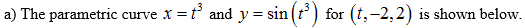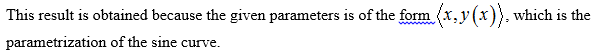# Plot the parametric curve x=t^3 ; y= sin(t^3); for (t, -2, 2).a) what familiar curve is produced? Why does the result happen? b) Give another parametrization for the same curve.

Question

Plot the parametric curve x=t^3 ; y= sin(t^3); for (t, -2, 2).

a) what familiar curve is produced? Why does the result happen?

b) Give another parametrization for the same curve.

check_circle

Step 1Step 2

Since the range of the curve is within –1 and 1, the curve obtained above is similar to the sine curve....

### Want to see the full answer?

See Solution

#### Want to see this answer and more?

Solutions are written by subject experts who are available 24/7. Questions are typically answered within 1 hour.*

See Solution
*Response times may vary by subject and question.
Tagged in

### Calculus